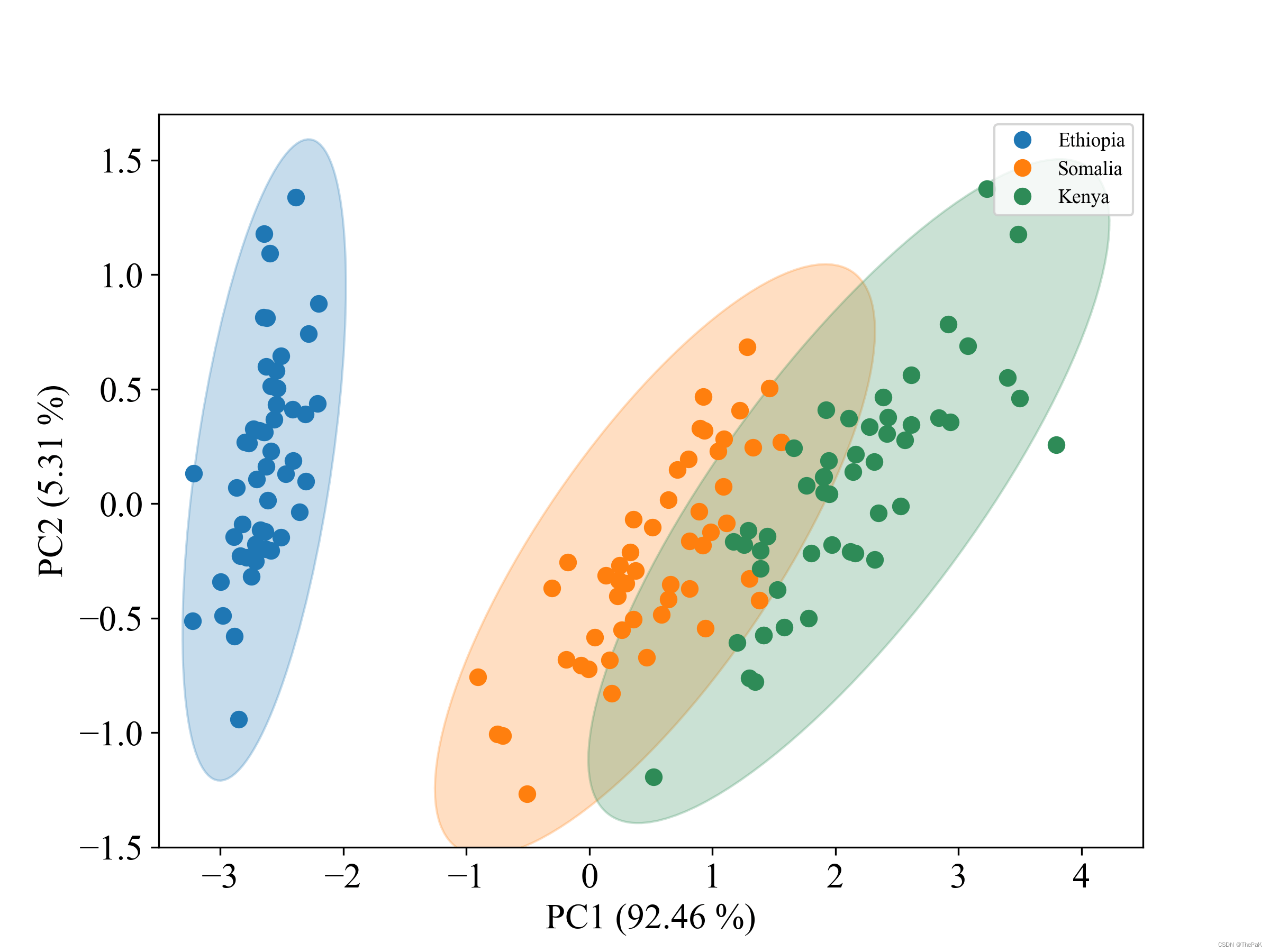# python实现PCA降维画分类散点图并标出95%的置信区间### 下面是完整代码：

``````from matplotlib.patches import Ellipse

def plot_point_cov(points, nstd=3, ax=None, **kwargs):
# 求所有点的均值作为置信圆的圆心
pos = points.mean(axis=0)
# 求协方差
cov = np.cov(points, rowvar=False)

return plot_cov_ellipse(cov, pos, nstd, ax, **kwargs)

def plot_cov_ellipse(cov, pos, nstd=3, ax=None, **kwargs):
def eigsorted(cov):
cov = np.array(cov)
vals, vecs = np.linalg.eigh(cov)
order = vals.argsort()[::-1]
return vals[order], vecs[:, order]
if ax is None:
ax = plt.gca()
vals, vecs = eigsorted(cov)

theta = np.degrees(np.arctan2(*vecs[:, 0][::-1]))
width, height = 2 * nstd * np.sqrt(vals)
ellip = Ellipse(xy=pos, width=width, height=height, angle=theta, **kwargs)
return ellip

'''画置信圆'''
def show_ellipse(X_pca, y, pca, flag=1):
# 定义颜色

colors = ['tab:blue', 'tab:orange', 'seagreen']
regions = ['Ethiopia', 'Somalia', 'Kenya']

# 定义分辨率
plt.figure(dpi=300, figsize=(8, 6))
# 三分类则为3
for i in range(0, 3):
pts = X_pca[y == int(i), :]
new_x, new_y = X_pca[y==i, 0], X_pca[y==i, 1]

plt.plot(new_x, new_y, '.', color=colors[i], label=regions[i], markersize=14)

plot_point_cov(pts, nstd=3, alpha=0.25, color=colors[i])

# 添加坐标轴
plt.xlim(-3.5, 4.5)
plt.ylim(-1.5, 1.7)
plt.xticks(size=16, family='Times New Roman')
plt.yticks(size=16, family='Times New Roman')
font = {'family': 'Times New Roman', 'size': 16}
plt.xlabel('PC1 ({} %)'.format(round(pca.explained_variance_ratio_ * 100, 2)), font)
plt.ylabel('PC2 ({} %)'.format(round(pca.explained_variance_ratio_ * 100, 2)), font)

plt.legend(prop={"family": "Times New Roman", "size": 9},  loc='upper right')
plt.show()

import pandas as pd
from sklearn.decomposition import PCA
import numpy as np
import matplotlib.pyplot as plt
labels = ['setosa', 'versicolor', 'virginica']
X = iris.data
y = iris.target_names[iris.target]

print("y length--------", len(y))
y_category = pd.Categorical(y,ordered=True,categories=['setosa', 'versicolor', 'virginica'])

y = y_category.codes
print(y)
print(y.shape)
print(type(y))
n_components = 2
pca = PCA(n_components=n_components)
X_pca = pca.fit_transform(X)
show_ellipse(X_pca, y, pca)``````

``````import numpy as np
import matplotlib.pyplot as plt
from matplotlib.patches import Ellipse
``````

`代码中的cov变量`是用于计算数据集协方差矩阵的。协方差矩阵用于描述多个变量之间的关系，其中对角线上的元素表示每个变量本身的方差，非对角线上的元素表示不同变量之间的协方差。

`np.degrees()` `该函数使用的公式：角度=弧度*180/pi`

`np.degrees()` 函数的参数是 `np.arctan2(*vecs[:, 0][::-1])`，它表示计算向量 `vecs` 中第一列（即下标为 0 的列）的极角（即与 x 轴的夹角）并将结果转换为角度制。

`vals, vecs = eigsorted(cov)`其目的是计算输入协方差矩阵的特征值和特征向量并返回排好序的结果。

`plot_cov_ellipse()`函数中，`width``height`分别表示绘制的椭圆的宽度和高度。具体来说，它们是通过将椭圆的半长轴和半短轴的长度设置为：

• 半长轴的长度等于`2 * nstd * sqrt(eigenvalue_1)`，其中`eigenvalue_1`是协方差矩阵的第一个特征值。
• 半短轴的长度等于`2 * nstd * sqrt(eigenvalue_2)`，其中`eigenvalue_2`是协方差矩阵的第二个特征值。
• 因此，`width``height`定义了椭圆的大小和形状，这取决于协方差矩阵的特征值以及`nstd`的值。当`nstd=1`时，它们定义了一个标准偏差的椭圆，绘制的置信椭圆包含了数据的约68.27%的置信区间。

`nstd=2` 表示椭圆的长度和宽度分别扩展到 2 倍标准差的大小，覆盖了大约 95.45% 的数据。这是因为在正态分布中，大约有 95.45% 的数据落在距离均值两个标准差的范围内。因此，使用 `nstd=2` 绘制置信椭圆通常被认为是覆盖 95% 的置信区间。

`nstd=3` 表示椭圆的长度和宽度分别扩展到 3 倍标准差的大小，覆盖了大约 99.73% 的数据。这是因为在正态分布中，大约有 99.73% 的数据落在距离均值三个标准差的范围内。因此，使用 `nstd=3` 绘制置信椭圆通常被认为是覆盖 99% 的置信区间。但需要注意的是，这里所提到的百分比是基于正态分布的假设。如果数据不符合正态分布，那么覆盖的百分比可能会有所不同。因此，对于非正态分布的数据，确定置信区间的百分比需要使用其他方法。

``````def plot_point_cov(points, nstd=3, ax=None, **kwargs):
# 求所有点的均值作为置信圆的圆心
pos = points.mean(axis=0)
# 求协方差矩阵
cov = np.cov(points, rowvar=False)

return plot_cov_ellipse(cov, pos, nstd, ax, **kwargs)

def plot_cov_ellipse(cov, pos, nstd=3, ax=None, **kwargs):
def eigsorted(cov):
cov = np.array(cov)
vals, vecs = np.linalg.eigh(cov)
order = vals.argsort()[::-1]
return vals[order], vecs[:, order]

if ax is None:
ax = plt.gca()
vals, vecs = eigsorted(cov)

theta = np.degrees(np.arctan2(*vecs[:, 0][::-1]))
width, height = 2 * nstd * np.sqrt(vals)
ellip = Ellipse(xy=pos, width=width, height=height, angle=theta, **kwargs)
return ellip
``````

最后，定义了一个函数 `show_ellipse()`，用于绘制样本点和置信椭圆。

``````'''画置信圆'''
def show_ellipse(X_pca, y, pca, flag=1):
# 定义颜色
colors = ['tab:blue', 'tab:orange', 'seagreen']
regions = ['Ethiopia', 'Somalia', 'Kenya']

# 定义分辨率
plt.figure(dpi=300, figsize=(8, 6))
# 三分类则为3
for i in range(0, 3):
pts = X_pca[y == int(i), :]
new_x, new_y = X_pca[y==i, 0], X_pca[y==i, 1]

plt.plot(new_x, new_y, '.', color=colors[i], label=regions[i], markersize=14)

plot_point_cov(pts, nstd=3, alpha=0.25, color=colors[i])

# 添加坐标轴
plt.xlim(-3.5, 4.5)
plt.ylim(-1.5, 1.7)
plt.xticks(size=16, family='Times New Roman')
plt.yticks(size=16, family='Times New Roman')
font = {'family': 'Times New Roman', 'size': 16}
plt.xlabel('PC1 ({} %)'.format(round(pca.explained_variance_ratio_ * 100, 2)), font)
plt.ylabel('PC2 ({} %)'.format(round(pca.explained_variance_ratio_ * 100, 2)), font)

plt.legend(prop={"family": "Times New Roman", "size": 9},  loc='upper right')
plt.show()``````

以数据集鸢尾花为例进行PCA降维，并使用上面代码画置信圆。

``````import pandas as pd
from sklearn.decomposition import PCA
import numpy as np
import matplotlib.pyplot as plt
labels = ['setosa', 'versicolor', 'virginica']
X = iris.data
y = iris.target_names[iris.target]

print("y length--------", len(y))
y_category = pd.Categorical(y,ordered=True,categories=['setosa', 'versicolor', 'virginica'])

y = y_category.codes
print(y)
print(y.shape)
print(type(y))
n_components = 2
pca = PCA(n_components=n_components)
X_pca = pca.fit_transform(X)
show_ellipse(X_pca, y, pca)``````

上面解析均为学习使用，如有问题请指正。